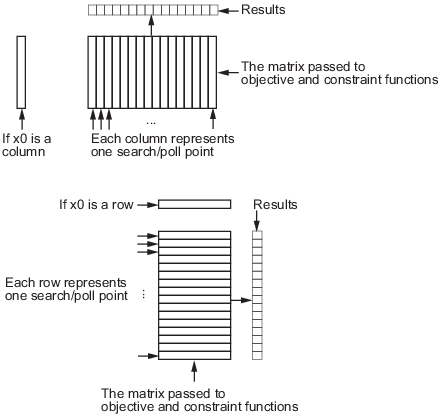## Vectorize the Objective and Constraint Functions

### Vectorize for Speed

Direct search often runs faster if you vectorize the objective and nonlinear constraint functions. This means your functions evaluate all the points in a poll or search pattern at once, with one function call, without having to loop through the points one at a time. Therefore, the option `UseVectorized` = `true` works only when `UseCompletePoll` or `UseCompleteSearch` is also set to `true`. However, when you set `UseVectorized` = `true`, `patternsearch` checks that the objective and any nonlinear constraint functions give outputs of the correct shape for vectorized calculations, regardless of the setting of the `UseCompletePoll` or `UseCompleteSearch` options.

If there are nonlinear constraints, the objective function and the nonlinear constraints all need to be vectorized in order for the algorithm to compute in a vectorized manner.

Note

Write your vectorized objective function or nonlinear constraint function to accept a matrix with an arbitrary number of points. `patternsearch` sometimes evaluates a single point even during a vectorized calculation.

### Vectorized Objective Function

A vectorized objective function accepts a matrix as input and generates a vector of function values, where each function value corresponds to one row or column of the input matrix. `patternsearch` resolves the ambiguity in whether the rows or columns of the matrix represent the points of a pattern as follows. Suppose the input matrix has `m` rows and `n` columns:

• If the initial point `x0` is a column vector of size `m`, the objective function takes each column of the matrix as a point in the pattern and returns a row vector of size `n`.

• If the initial point `x0` is a row vector of size `n`, the objective function takes each row of the matrix as a point in the pattern and returns a column vector of size `m`.

• If the initial point `x0` is a scalar, `patternsearch` assumes that `x0` is a row vector. Therefore, the input matrix has one column (`n` = 1, the input matrix is a vector), and each entry of the matrix represents one row for the objective function to evaluate. The output of the objective function in this case is a column vector of size `m`.

Pictorially, the matrix and calculation are represented by the following figure.

Structure of Vectorized FunctionsFor example, suppose the objective function is

`$f\left(x\right)={x}_{1}^{4}+{x}_{2}^{4}-4{x}_{1}^{2}-2{x}_{2}^{2}+3{x}_{1}-{x}_{2}/2.$`

If the initial vector `x0` is a column vector, such as `[0;0]`, a function for vectorized evaluation is

```function f = vectorizedc(x) f = x(1,:).^4+x(2,:).^4-4*x(1,:).^2-2*x(2,:).^2 ... +3*x(1,:)-.5*x(2,:);```
If the initial vector `x0` is a row vector, such as `[0,0]`, a function for vectorized evaluation is
```function f = vectorizedr(x) f = x(:,1).^4+x(:,2).^4-4*x(:,1).^2-2*x(:,2).^2 ... +3*x(:,1)-.5*x(:,2);```

Tip

If you want to use the same objective (fitness) function for both pattern search and genetic algorithm, write your function to have the points represented by row vectors, and write `x0` as a row vector. The genetic algorithm always takes individuals as the rows of a matrix. This was a design decision—the genetic algorithm does not require a user-supplied population, so needs to have a default format.

To minimize `vectorizedc`, enter the following commands:

```options=optimoptions('patternsearch','UseVectorized',true,'UseCompletePoll',true); x0=[0;0]; [x,fval]=patternsearch(@vectorizedc,x0,... [],[],[],[],[],[],[],options)```

MATLAB® returns the following output:

```Optimization terminated: mesh size less than options.MeshTolerance. x = -1.5737 1.0575 fval = -10.0088```

### Vectorized Constraint Functions

Only nonlinear constraints need to be vectorized; bounds and linear constraints are handled automatically. If there are nonlinear constraints, the objective function and the nonlinear constraints all need to be vectorized in order for the algorithm to compute in a vectorized manner.

The same considerations hold for constraint functions as for objective functions: the initial point `x0` determines the type of points (row or column vectors) in the poll or search. If the initial point is a row vector of size k, the matrix x passed to the constraint function has k columns. Similarly, if the initial point is a column vector of size k, the matrix of poll or search points has k rows. The figure Structure of Vectorized Functions may make this clear. If the initial point is a scalar, `patternsearch` assumes that it is a row vector.

Your nonlinear constraint function returns two matrices, one for inequality constraints, and one for equality constraints. Suppose there are nc nonlinear inequality constraints and nceq nonlinear equality constraints. For row vector `x0`, the constraint matrices have nc and nceq columns respectively, and the number of rows is the same as in the input matrix. Similarly, for a column vector `x0`, the constraint matrices have nc and nceq rows respectively, and the number of columns is the same as in the input matrix. In figure Structure of Vectorized Functions, “Results” includes both nc and nceq.

### Example of Vectorized Objective and Constraints

Suppose that the nonlinear constraints are

Write a function for these constraints for row-form `x0` as follows:

```function [c ceq] = ellipsecosh(x) c(:,1)=x(:,1).^2/9+x(:,2).^2/4-1; c(:,2)=cosh(x(:,1))-x(:,2)-1; ceq=[];```

Minimize `vectorizedr` (defined in Vectorized Objective Function) subject to the constraints `ellipsecosh`:

```x0=[0,0]; options = optimoptions('patternsearch','UseVectorized',true,'UseCompletePoll',true); [x,fval] = patternsearch(@vectorizedr,x0,... [],[],[],[],[],[],@ellipsecosh,options)```

MATLAB returns the following output:

```Optimization terminated: mesh size less than options.MeshTolerance and constraint violation is less than options.ConstraintTolerance. x = -1.3516 1.0612 fval = -9.5394```